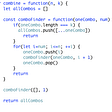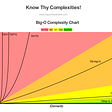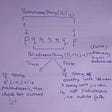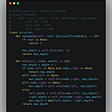# Algorithm

1252. Cells with Odd Values in a Matrix

https://leetcode.com/problems/cells-with-odd-values-in-a-matrix/

Given `n` and `m` which are the dimensions of a matrix initialized by zeros and given an array `indices` where `indices[i] = [ri, ci]`. For each pair of `[ri, ci]` you have to increment all cells in row `ri` and column `ci` by 1.

Return the number of cells with odd values in the matrix after applying the increment to all `indices`.

Example 1:

`Input: n = 2, m = 3, indices = [[0,1],[1,1]]Output: 6Explanation: Initial matrix = [[0,0,0],[0,0,0]].After applying first increment it becomes [[1,2,1],[0,1,0]].The final matrix will be [[1,3,1],[1,3,1]] which contains 6 odd numbers.`

Example 2:

`Input: n = 2, m = 2, indices = [[1,1],[0,0]]Output: 0Explanation: Final matrix = [[2,2],[2,2]]. There is no odd number in the final matrix.`

Constraints:

• `1 <= n <= 50`
• `1 <= m <= 50`
• `1 <= indices.length <= 100`
• `0 <= indices[i] < n`
• `0 <= indices[i] < m`

1572. Matrix Diagonal Sum

https://leetcode.com/problems/matrix-diagonal-sum/

Given a square matrix `mat`, return the sum of the matrix diagonals.

Only include the sum of all the elements on the primary diagonal and all the elements on the secondary diagonal that are not part of the primary diagonal.

Example 1:

`Input: mat = [[1,2,3],              [4,5,6],              [7,8,9]]Output: 25Explanation: Diagonals sum: 1 + 5 + 9 + 3 + 7 = 25Notice that element mat = 5 is counted only once.`

Example 2:

`Input: mat = [[1,1,1,1],              [1,1,1,1],              [1,1,1,1],              [1,1,1,1]]Output: 8`

Example 3:

`Input: mat = []Output: 5`

Constraints:

• `n == mat.length == mat[i].length`
• `1 <= n <= 100`
• `1 <= mat[i][j] <= 100`

1323. Maximum 69 Number

https://leetcode.com/problems/maximum-69-number/

Given a positive integer `num` consisting only of digits 6 and 9.

Return the maximum number you can get by changing at most one digit (6 becomes 9, and 9 becomes 6).

Example 1:

`Input: num = 9669Output: 9969Explanation: Changing the first digit results in 6669.Changing the second digit results in 9969.Changing the third digit results in 9699.Changing the fourth digit results in 9666. The maximum number is 9969.`

Example 2:

`Input: num = 9996Output: 9999Explanation: Changing the last digit 6 to 9 results in the maximum number.`

Example 3:

`Input: num = 9999Output: 9999Explanation: It is better not to apply any change.`

Constraints:

• `1 <= num <= 10^4`
• `num`'s digits are 6 or 9.

832. Flipping an Image

https://leetcode.com/problems/flipping-an-image/

Given a binary matrix `A`, we want to flip the image horizontally, then invert it, and return the resulting image.

To flip an image horizontally means that each row of the image is reversed. For example, flipping `[1, 1, 0]` horizontally results in `[0, 1, 1]`.

To invert an image means that each `0` is replaced by `1`, and each `1` is replaced by `0`. For example, inverting `[0, 1, 1]` results in `[1, 0, 0]`.

Example 1:

`Input: [[1,1,0],[1,0,1],[0,0,0]]Output: [[1,0,0],[0,1,0],[1,1,1]]Explanation: First reverse each row: [[0,1,1],[1,0,1],[0,0,0]].Then, invert the image: [[1,0,0],[0,1,0],[1,1,1]]`

Example 2:

`Input: [[1,1,0,0],[1,0,0,1],[0,1,1,1],[1,0,1,0]]Output: [[1,1,0,0],[0,1,1,0],[0,0,0,1],[1,0,1,0]]Explanation: First reverse each row: [[0,0,1,1],[1,0,0,1],[1,1,1,0],[0,1,0,1]].Then invert the image: [[1,1,0,0],[0,1,1,0],[0,0,0,1],[1,0,1,0]]`

Notes:

• `1 <= A.length = A.length <= 20`
• `0 <= A[i][j] <= 1`

--

--

--

## More from Joo Hee Paige Kim

Consistency achieves everything https://github.com/paigekim29

Love podcasts or audiobooks? Learn on the go with our new app.

## Provisioning Kubernetes on top of AWS with the help of Ansible.## Is it Worth Switching to Linux?## Making Our Elasticsearch Indices More Manageable## Introduction to my game dev blog## Print to “PRE-PRINTED” set of forms using Jasper Reports without a Database, in Java## What makes a technical architect?## How to Debug Xamarin Application on Visual Studio## Joo Hee Paige Kim

Consistency achieves everything https://github.com/paigekim29

## Algorithms: Recursion/Backtracking, Combinations## Understanding (luv’n) JavaScript thru Data Structures & Algorithms (DS & A)## Mastering Recursion For Beginners-(Part 3)## LeetCode Patterns Adventure 24 — Maximum Depth of Binary Tree